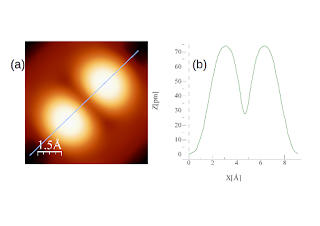## Pages

### Codes

The codes developed in the group are hosted in GitHub.

1. STM images, conductance plots and dI/dV maps.-

Our awesome Tersoff and Hamann (TH) and Bardeen code based on planewave DFT calculations: STMpw.f90, you can download it from here: https://github.com/qphensurf/STMpw/releases

Summary of STMpw.f90 :
The present version runs on converged results of the VASP code. It needs to have the POSCAR, OUTCAR and WAVECAR of the VASP run that has been converged with the option ISYM=0.

The code produces constant-current images as well as single-point dI/dV curves and dI/dV maps. The actual agreement between simulations and experiments is sometimes breathtaking, particularly after all the bashing that is usually done on DFT calculations.

A big problem of STM simulations based on supercell codes (typically planewave codes that use FFT in 3D) is the vacuum region. In order to simulate this region next to the surface of a slab mimicking the system of interest, a small vacuum region is usually left. This makes totally sense because wavefunctions are exponentially decaying in vacuum and it is maddening to try to reproduce them with finite sets of planewaves. A small vacuum region implies less planewaves because the density is never so small that a ridiculous number of planewave is needed. But this is bad when you are simulating STM images made by the tails of these wavefunctions in vacuum. Usually, you need the value of the wavefunction where the density coming from the ''back'' of the slab (that you do not want to have) is larger!

STMpw goes about this problem by realizing that each Fourier component of the wavefunction abides by a Schrödinger equation that is trivially solvable if the electron potential is constant. We can then write the ''exact'' solution of the wavefunction in the vacuum region that needs to match the correct DFT wavefunction in the slab. To do this we define a plane at a distance z_s from the surface where the slab solutions and the vacuum solutions are matched. The user only needs to give the code this distance, and the code runs. The distance should be chosen there where the density is still large enough to be accurate, but where it already corresponds to the vacuum solution. Our experience is that 2 Angströms beyond the last atom is excellent.

Once that we know the exact values of the wavefunctions there where the STM tip "lives", we can evaluate the STM image. To do this we apply either the Tersoff and Hamann approximation in its finite-bias extension (à la Norton Lang) or we do a full-fledged Bardeen approximation.Figure: Test included in the distribution of STMpw.f90 (GitHub): This is an acetylene molecule (HCCH for the physicist among you) on a Cu (100) surface. This is a very simplified calculation where a 3x3 surface unit cell was used, 4 atomic layers to create the slab. The full cell is 7.524x7.524x21.282 Angströms^3 where the vacuum gap is 7.835 Angströms. The image has been produced using the Tersoff-Hamman approach integrating 0.5 eV from the Fermi energy. This would correspond to an image taken at 0.5 V, assuming that the Kohn-Sham eigenergies of the DFT calculation correspond to the one-electron energies of the experiment.  The image (a) and the cut has been produced at a largish constant integrated local density of states, and the tip is fairly close to the molecule, but always above 2.5 Angströms from the H atoms. (b) is the profile along the cut plotted in (a). The image has been produced in a linux machine running wine and WSxM. A .prn file was saved and read using gimp to produce the above image.